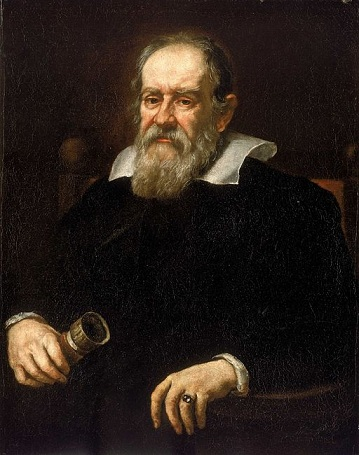# What is the Relationship Between Mass and Inertia

## Mass and Inertia

The terms mass and inertia are both important concepts in classical as well as modern physics. The relationship between mass and inertia is that inertia is a term that qualitatively describes the ability of a substance to resist changes in its state of motion, while mass gives a quantitative value for inertia. However, the term mass is not only used to quantify inertia but also to quantify other physical properties of a substance as well.

## What is Inertia

Inertia is a term that qualitatively describes an object’s reluctance to change its state of motion. Due to inertia:

• An object at rest tends to stay at rest and,
• An object in motion tends to move at constant speed in a straight line.

This means that a force needs to be applied to move a body at rest, or to change the speed or direction of motion of a body already in motion.

The concept of inertia as it is known today was developed by Galileo. Prior to him, most people believed that the “natural state” of a body is to stay at rest. They argued that when an object rolls along the ground, for instance, it eventually comes to a stop because it is trying to reach the “natural state” of rest. However Galileo, and subsequently Newton, argued that in this case the object comes to a rest because there are forces that oppose the object, while the object itself is trying to maintain its state of motion.Galileo Galilei was a pioneer in developing the ideas about inertia in classical physics.

## What is Mass

Mass has several different descriptions in physics, and in one of these descriptions, mass is a quantitative measurement of inertia. The more mass that an object has, the more difficult it is for a force to change the object’s state of motion (when we say “state of motion” here, that includes the “state of rest” as well). When mass is used as a measurement of inertia, it is called inertial mass. In classical physics, the concept of inertial mass comes up in Newton’s second law of motion.

According to Newton’s second law, if a resultant force$\Sigma F$ acts on an object of mass$m$, it would give an acceleration$a$ to the object in the direction of force. These quantities are related by:$\Sigma F=ma$

An acceleration is a change in an object’s state of motion. According to this formula, a larger force is needed to give the same acceleration to a body having a larger mass. So here, mass is the quantity that resists changes to the body’s state of motion, and therefore, mass is a measurement of inertia.

However, mass is also used in another context: to quantify forces of gravitational attraction between objects. In this sense, the term active gravitational mass refers to how strong a gravitational field that an object can produce. The term passive gravitational mass describes how strongly an object interacts with the gravitational field set up by another object. In classical physics, the values of “mass” used in Newton’s law of gravitation are these gravitational masses. Although the meaning of mass is conceptually different under these two contexts, according to the principle of equivalence in general relativity, gravitational and inertial masses of objects are equivalent. Experimentally, the equivalence between gravitational and inertial mass has been confirmed to a high accuracy of 5 parts in 1014 .

## What is the relationship between Mass and Inertia

Inertia is a qualitative description which describes an ability of an object to resist changes to its state of motion.

Mass is a physical quantity that indicates an object’s inertia. It is quantitative.

Mass describes not only an object’s ability to resist changes to its state of motion, but also how objects interact with gravitational forces. Technically, inertia is not concerned with how an object interacts with gravitational forces. However, it appears that an object’s ability to resist changes in motion and its ability to interact via gravity are quantitatively equivalent.

References

1. O’Donnell, P. J. (2015). Essential Dynamics and Relativity. Taylor & Francis.

Image Courtesy

“Portrait of Galileo Galilei” by Justus Susterman (1597-1681) [Public Domain], via Wikimedia Commons